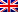# Resolution Assignment with Excel

Contenus

## Solving assignment problem with Excel

Here's how to troubleshoot assignment problems with Excel.

To formulate an assignment problem, it is necessary to answer these three questions.

• What are the decisions to be made? For this problem, we need Excel to know which person to assign to which task (Yes = 1, No = 0). For example, if we assign person 1 to task 1, cell C10 is equal to 1. Otherwise, cell C10 is equal to 0. (in yellow)
• What are the constraints on these decisions? Each person can only perform one task (Supply = 1). Each task only needs one person (Request = 1). (in light blue)
• What is the overall measure of performance for these decisions? The overall measure of performance is the total cost of the assignment. The objective is therefore to minimize this quantity. (in dark blue)

Let us name the following ranges:

And let's insert the following functions:

## Solve the model

Let’s enter the solver parameters:

The optimal solution is:FRFRENES